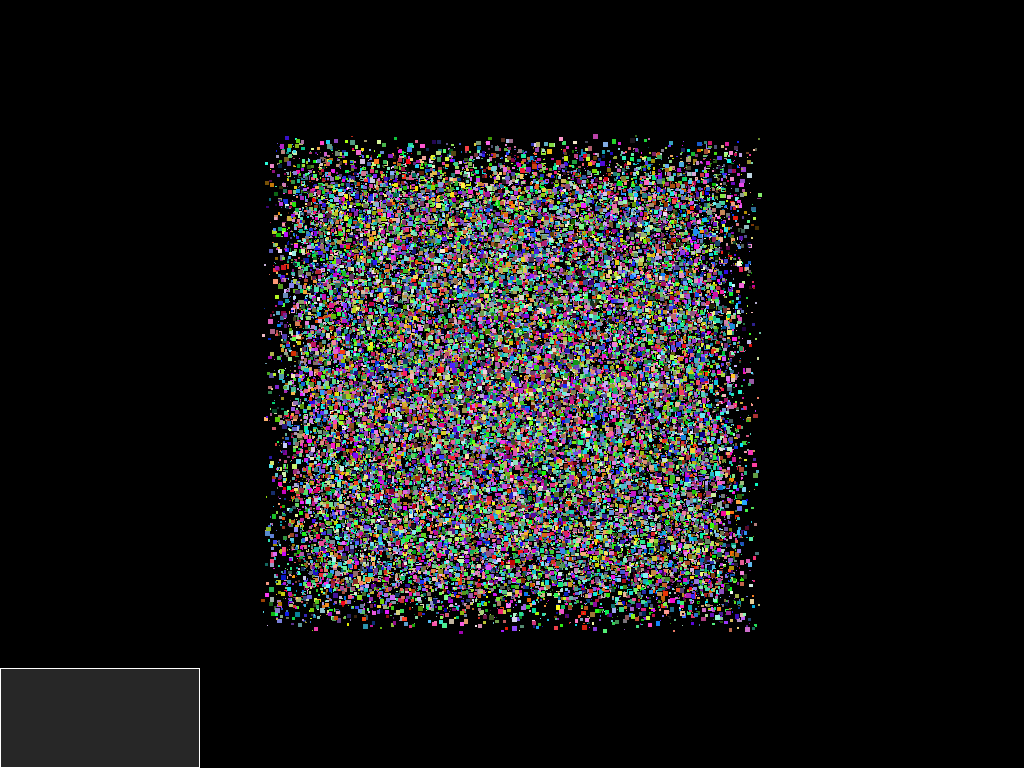# Selecting multiple objects#

Here we show how to select objects in the 3D world. In this example all objects to be picked are part of a single actor.

FURY likes to bundle objects in a few actors to reduce code and increase speed. Nonetheless the method works for multiple actors too.

The difference with the picking tutorial is that here we will be able to select more than one object. In addition we can select interactively many vertices or faces.

In summary, we will create an actor with thousands of cubes and then interactively we will be moving a rectangular box by hovering the mouse and making transparent everything that is behind that box.

```import numpy as np

from fury import actor, pick, utils, window
```

Adding many cubes of different sizes and colors

```num_cubes = 50000

centers = 10000 * (np.random.rand(num_cubes, 3) - 0.5)
colors = np.random.rand(num_cubes, 4)
colors[:, 3] = 1.0
radii = 100 * np.random.rand(num_cubes) + 0.1
```

Keep track of total number of triangle faces Note that every quad of each cube has 2 triangles and each cube has 6 quads in total.

```num_faces = num_cubes * 6 * 2
```

Build scene and add an actor with many objects.

```scene = window.Scene()
```

Build the actor containing all the cubes

```cube_actor = actor.cube(centers, directions=(1, 0, 0), colors=colors, scales=radii)
```

Access the memory of the vertices of all the cubes

```vertices = utils.vertices_from_actor(cube_actor)
num_vertices = vertices.shape
num_objects = centers.shape
```

Access the memory of the colors of all the cubes

```vcolors = utils.colors_from_actor(cube_actor, 'colors')
```

Create a rectangular 2d box as a texture

```rgba = 255 * np.ones((100, 200, 4))
rgba[1:-1, 1:-1] = np.zeros((98, 198, 4)) + 100
texa = actor.texture_2d(rgba.astype(np.uint8))

scene.reset_camera()
scene.zoom(3.0)
```

Create the Selection Manager

```selm = pick.SelectionManager(select='faces')
```

Tell Selection Manager to avoid selecting specific actors

```selm.selectable_off(texa)
```

Let’s make the callback which will be called when we hover the mouse

```def hover_callback(_obj, _event):
event_pos = selm.event_position(showm.iren)
# updates rectangular box around mouse
texa.SetPosition(event_pos - 200 // 2, event_pos - 100 // 2)

# defines selection region and returns information from selected objects
info = selm.select(event_pos, showm.scene, (200 // 2, 100 // 2))
for node in info.keys():
if info[node]['face'] is not None:
if info[node]['actor'] is cube_actor:
for face_index in info[node]['face']:
# generates an object_index to help with coloring
# by dividing by the number of faces of each cube (6 * 2)
object_index = face_index // 12
sec = int(num_vertices / num_objects)
color_change = np.array([150, 0, 0, 255], dtype='uint8')
vcolors[
object_index * sec : object_index * sec + sec
] = color_change
utils.update_actor(cube_actor)
showm.render()
```

Make the window appear

```showm = window.ShowManager(
scene, size=(1024, 768), order_transparent=True, reset_camera=False
)
```

Bind the callback to the actor

```showm.add_iren_callback(hover_callback)
```

Change interactive to True to start interacting with the scene

```interactive = False

if interactive:

showm.start()
```

Save the current framebuffer in a PNG file

```window.record(showm.scene, size=(1024, 768), out_path='viz_selection.png')
```Total running time of the script: ( 0 minutes 2.520 seconds)

Gallery generated by Sphinx-Gallery